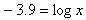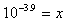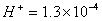pH
Examples

Example 1 Find the pH of apple juice with a hydrogen ion concentration of 3.2 x 10-4 .

Step 1. Substitute 3.2 x 10-4 in the formula.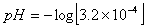Step 2. Use a calculator to solve.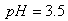Example 2
Find the hydrogen ion concentration of ketchup which has a pH of 3.9.

Step 1. Substitute 3.9 into the formula.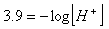Step 2. Use the inverse operations of logs and exponents and a calculator to solve.